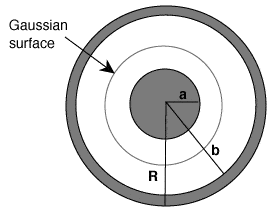Flux Through a Gaussian Surface

Homework Statement

A metal sphere of radius a is surrounded by a metal shell of inner radius b and outer radius R, as shown in the diagram below. The flux through a spherical Gaussian surface located between a and b is 1.20Q/εo and the flux through a spherical Gaussian surface just outside R is 0.80Q/εo.
a) What is the total charge on the inner sphere? (Express your answer as a multiple of Q. For example, if the total charge is 0.2Q, then input 0.2).Homework Equations

flux=E4pi*r^2=Qenclosed/epsilon not

The Attempt at a Solution

I understand that I need to use the ratios of 1.2Q/Enot=Qenclosed/Enot and .8Q/Enot=Qenclosed/Enot
I'm just not sure what to do with these two things

Delta2
Homework Helper
Gold Member
Sorry for this question but do you understand what the meaning of ##Q_{enclosed}## is? If yes you should be able to see that the ##Q_{enclosed}## by the Gaussian surface given by the problem is equal to the charge of the metal sphere of radius a. And using the first of ratios you wrote you ll easily conclude that ##Q_{enclosed}## is equal to ...

haruspex
Homework Helper
Gold Member
epsilon not
It's "epsilon nought". I've heard video tutorials, from India I think, which pronounce it "not" instead of "nought".

Sorry for this question but do you understand what the meaning of ##Q_{enclosed}## is? If yes you should be able to see that the ##Q_{enclosed}## by the Gaussian surface given by the problem is equal to the charge of the metal sphere of radius a. And using the first of ratios you wrote you ll easily conclude that ##Q_{enclosed}## is equal to ...
equal to what?

Delta2
Homework Helper
Gold Member
equal to what?
1.2Q of course!!!

•Steve4Physics
Steve4Physics
Homework Helper
Gold Member
Hi, Suppose a surface completely surrounds a charge of, say, 3C. What is the total flux passing through the surface?

Use Gauss's law to answer the question. If you can't do it, you might need to do a little reading or watch a YouTube video first.

After that you might be able to answer the original question. Or we'll be in a better position to help you.

1.2Q of course!!!
wouldn't it be -1.2Q? not 1.2Q?

Steve4Physics
Homework Helper
Gold Member
wouldn't it be -1.2Q? not 1.2Q?
•